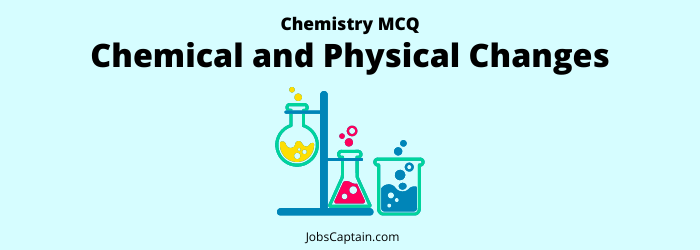# Chemical and Physical Changes MCQ Quiz: ChemistryThis Chemistry Chemical and Physical Changes MCQ Quiz is designed to test your knowledge on the topic. The quiz consists of 18 multiple choice questions, each with 4 possible answers. In this test, you will find the most important and frequently asked questions about chemistry chemical and physical changes.

Question 1. Pasteurization is related to?

(A) Distillation of milk

(B) Fermentation of milk

(C) Dehydration of milk

(D) Sterilization of milk

(D) Sterilization of milk

Question 2. Which of the following statement is not true?

(A) When a solution is separated with the help of semipermeable membrane, then water flow can be stopped by giving pressure to the solution

(B) Water vapour pressure of any solution is lower than vapour pressure of pure water

(C) Addition of solute to a solution increases its water potential

(D) Boiling point of any water solution is higher than pure water

(C) Addition of solute to a solution increases its water potentialn

Question 3. Because of impurities the boiling point of a liquid?

(A) They are not related

(B) Remains constant

(C) Decreases

(D) Increases

(D) Increases

Question 4. Why does water boil at a temperature below 100ºC at higher altitudes?

(A) None of the above is correct

(B) Because of heavy winds in mountains

(C) The gravitational attraction is less

(D) The atmospheric pressure decreases and hence boiling point is lowered

(D) The atmospheric pressure decreases and hence boiling point is lowered

Question 5. Which type of colloidal system is expressed in the fog?

(A) Liquid in liquid

(B) Solid in a gas

(C) Gas in a liquid

(D) Liquid in a gas

(D) Liquid in a gas

Question 6. The technique of chromatography is used to ______.

(A) Separate the substances from a mixture

(B) Dry distillation of colouring substances

(C) Determine the structure of substances

(D) Identify colour substances

(A) Separate the substances from a mixture

Question 7. Process of solid camphor into camphor vapour is called?

(A) Sublimation

(B) Melting

(C) Freezing

(D) Vaporisation

(A) Sublimation

Question 8. Example of corrosion is ________.

(A) Above three

(B) Brown coating on Iron

(C) Green coating on Copper

(D) Black coating on Silver

(A) Above three

Question 9. Which of the following is/are the example/examples of chemical change.

1. Crystallization of sodium chloride
2. Melting of ice
3. Souring of milk

Select the correct answer using the code given below

(A) 1, 2 and 3

(B) Only 3

(C) Only 1 and 2

(D) None of the above

(B) Only 3

Question 10.

• Assertion (A): A chemical reaction becomes faster at a higher temperature.
• Reason (R): At higher temperature, molecular motion becomes more rapid.

(A) (A) is false, but (R) is true

(B) (A) is true, but (R) is false

(C) Both (A) and (R) are true, but (R) is not the correct explanation of (A)

(D) Both (A) and (R) are true and (R) is the correct explanation of (A)

(D) Both (A) and (R) are true and (R) is the correct explanation of (A)

Question 11. Give a thought to these statements.

• Statement (A): Temperature below 0ºC is achieved by the mixture of salt and ice.
• Reason (R): Salt increases the freezing point of ice.

In the context of above statements which one of the following is correct?

(A) (A) is false, but (R) is true

(B) (A) is true, but (R) is false

(C) Both (A) and (R) are true, but (R) is not the correct explanation of (A)

(D) Both (A) and (R) are true and (R) is the correct explanation of (A)

(B) (A) is true, but (R) is false

Question 12. . Give thought to following statements.

1. In the process of osmosis, the solvent moves towards a dilute solution from the concentrated solution.
2. In reverse osmosis internal pressure but upon a dilute solution.

Among above statements which is/ are correct.

(A) Neither 1 nor 2

(B) Both 1 and 2

(C) Only 2

(D) Only 1

(A) Neither 1 nor 2

Question 13. Match List-I (Oxidation number) with List-II (the element) and select the correct answer using the code given below the lists.

 List-I List-II (a) 2 1. Oxidation number of Mn in MnO2 (b) 3 2. Oxidation number of S in H2 SO4 (c) 4 3. Oxidation number of Ca in CaO (d) 6 4. Oxidation number of Al in NaAlH4

Code

 (a) (b) (c) (d) (A) 4 3 2 1 (B) 3 4 2 1 (C) 4 3 1 2 (D) 3 4 1 2
(D) 3 4 1 2

Question 14. Pasteurization is a process by which?

(A) None of the above

(B) First milk is heated up to a longer time and then cooled suddenly within specified time

(C) Milk is heated for 8 hours

(D) Milk is preserved at a very low temperature for 24 hours

(B) First milk is heated up to a longer time and then cooled suddenly within specified time

Question 15. Change of water into the vapour is called?

(A) Biological

(B) Chemical

(C) Physical

(D) Natural

(C) Physical

Question 16. An example of physical change?

(A) Dissolving of sugar in water

(B) Making of curd from milk

(C) Burning of candle

(D) Blackening of silverware

(A) Dissolving of sugar in water

Question 17. What form of energy is produced in the decomposition of water?

(A) Acid

(B) Sound

(C) Heat

(D) Light

(C) Heat

Question 18. Which of the following is an example of chemical change?

(A) Dissolving of salt in water

(B) Wet soil lamp, when dried, get fragile

(C) Softening of vegetable when cooked

(D) Dispersion of white light into the light of 7 colours when passed through a prism